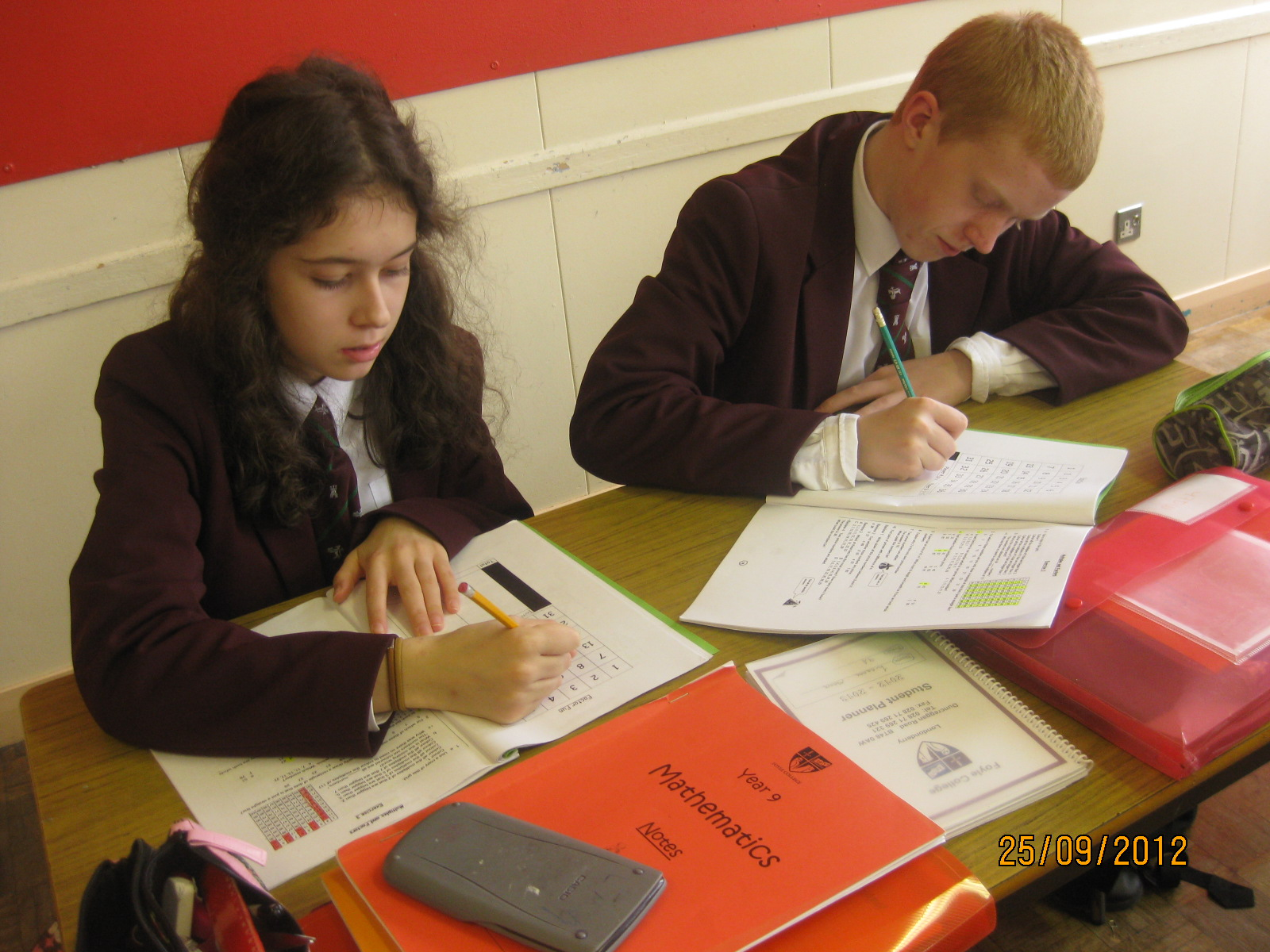# KS3 MathsIn Key Stage 3 Mathematics students will learn how to solve numerical problems and carry out calculations with and without the aid of a calculator. They will progress to forming and solving algebraic equations, dealing with formulae and plotting graphs. Students will explore the geometrical properties of 2D and 3D shapes and learn how to calculate their areas or volumes. They will collect and interpret statistical data and gain the skills required to calculate the probability of a situation in a real-life context occurring.

Students will learn that Mathematics is about more than all of the above; it is about thinking logically and being able to apply the principles they learn to everyday situations.

The Key Stage 3 Mathematics course at Foyle College begins by giving students the opportunity to review mathematical facts and techniques learnt in Primary School before expanding on these to begin the students’ preparation for GCSE Mathematics.

Course Details

The ‘Mathematics with Financial Capability’ area of learning of the Northern Ireland KS3 curriculum is mainly delivered through the subject of Mathematics.

Students in Years 8 – 10 have 5 periods of Mathematics per week.

Course Content

The topics covered by each KS3 year group are listed below.Coordinates Angle Pairs Dealing with Numbers Tilings & Symmetry Dealing with Numbers Distance and Direction Decimals, Fractions & Percentages Expressions and Formulas Personal Finance Expressions and Formulas Statistics Pythagoras' Theorem Angles Transformations Trigonometry Equations Probability Probability Dealing with Numbers Negative Numbers Equations and Inequalities Measurement Measurement Measurement Probability Decimals, Fractions & Percentages Statistics Statistics Equations and Inequalities Simultaneous Equations Sequences Ratio Speed, Distance and Time 2D Shapes Three Dimensions

Teaching and Learning

Students often find learning mathematics intimidating, but our aim is to guide them through the subject in easy steps, building both their understanding and their confidence.

Students will be taught using a combination of traditional methods, PowerPoint presentations, other ICT-based activities and practical investigations. This will cater for a range of different learning styles. Practice of the required mathematical techniques will be gained through a variety of written, oral and practical work, including the use of ICT.  Students will work independently or in groups.

Students will have access to comprehensive notes to assist with both their initial understanding of a concept and revision prior to assessment. These notes have also proved beneficial to parents keen to help their child with their work!

Year 9 pupils working on ‘Factor Fun